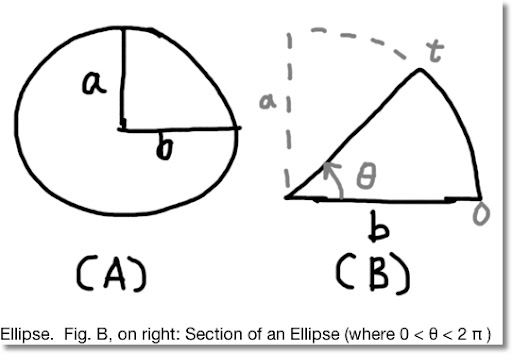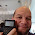## Wednesday, May 23, 2012

### Area of an EllipseBlog Entry #104. (I thought I use a different font this time. I like Arial, but change is good, at least every once in a while. )

Objective of this blog entry: calculate the area of an ellipse (Fig. A), and then a section of an ellipse (Fig. B).

Normally, the equation given for an ellipse is:

x^2/a^2 + y^2/b^2 = 1

I am going to use the parametric form

x = a cos t
y = b sin t
with 0 ≤ t ≤ 2 π

Let θ be the upper limit (angle) and the area is:

θ
∫ y dx =
0

θ
∫ y(t) x'(t) dt =
0

θ
∫ (b sin t) (a sin t) dt =
0

θ
∫ a b sin^2 t dt
0

Note: the antiderviatve of sin^2 t is 1/2 ( t - sin t cos t ).

Then:

θ
∫ a b sin^2 t dt =
0

a b [ 1/2 ( θ - sin θ cos θ ) - 1/2 ( 0 - sin 0 cos 0 ) ] =

1/2 a b ( θ - sin θ cos θ )

The area of an ellipse (up to angle θ , see Fig. B) is:
1/2 a b ( θ - sin θ cos θ )

To find the area of the entire ellipse, let θ = 2 π. Then:

A = 1/2 a b (2 π - sin (2 π) cos (2 π)) = π a b

That concludes is blog entry. Before I go, and before I forget: THANK YOU U.S. TROOPS FOR ALL YOU DO!!!!

Eddie

#### 3 comments:

1.Thanks for providing such useful information about ellipse .The formulas and their derivation which are provided here are very useful.
Area of an Ellipse

2.You are welcome Manoj.

3.### Swiss Micros DM16L: Advanced Boolean and Factorial (up to 20)

Swiss Micros DM16L:   Advanced Boolean and Factorial (up to 20) Introduction The program listing, for the Swiss Micros DM16L and Hewlett Pac...